# Matrices & Determinants – 1 – 1

1. A square matrix all of whose elements except the main diagonal are zeros is called a :

(a) null matrix
(b) singular matrix
(c) diagonal matrix
(d) symmetric matrix

Explanation
No answer description available for this question. Let us discuss.

2. Square matrix A for which AT = -A is called a :

(a) row matrix
(b) column matrix
(c) symmetric matrix
(d) skew-symmetric matrix

Explanation
No answer description available for this question. Let us discuss.

3. Square matrix A for which AT = A is called a :

(a) row matrix
(b) column matrix
(c) symmetric matrix
(d) skew-symmetric matrix

Explanation
No answer description available for this question. Let us discuss.

4. A square matrix A with complex entries for which (A)= –A is called :

(a) identity matrix
(b) hermitian matrix
(c) symmetric matrix
(d) skew-hermitian matrix

Explanation
No answer description available for this question. Let us discuss.

5. This matrix is a _______ .(a) null matrix
(b) row matrix
(c) square matrix
(d) symmetric matrix

Explanation
No answer description available for this question. Let us discuss.

General Knowledge Books##General Knowledge 2019 Price: Check on Amazon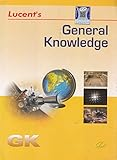-17% General Knowledge Price: INR 179.00 Was: INR 216.00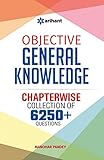-43% Objective General Knowledge Chapterwise Collection of 6250+ Questions Price: INR 169.00 Was: INR 295.00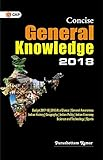Concise General Knowledge 2018 Price: INR 30.00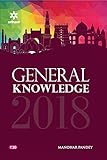General Knowledge 2018 (Old Edition) Price: Check on Amazon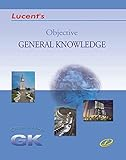-23% Objective General Knowledge Price: INR 302.00 Was: INR 390.00 ‹›

Be a Part of the New & Next

Share the Knowledge

Copy Protected by Chetan's WP-Copyprotect.